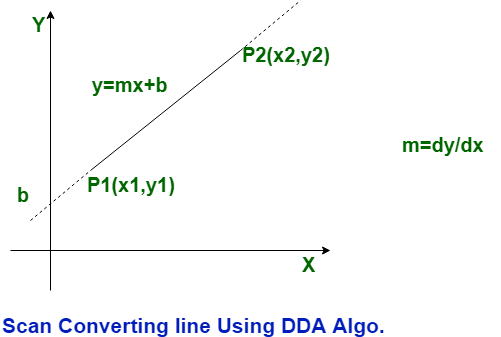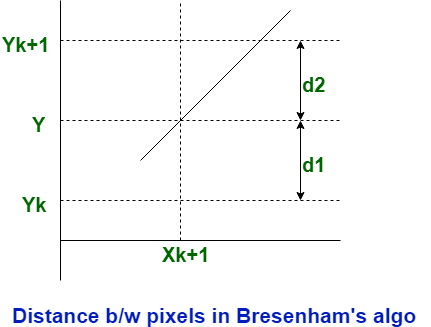# Comparions between DDA and Bresenham Line Drawing algorithm

• Difficulty Level : Hard
• Last Updated : 03 Jun, 2019

In lighting tricks, there are 2 algorithmic rules used for drawing a line over the screen that’s DDA stands for Digital Differential Analyser algorithmic rule and Bresenham line algorithm.The main distinction between DDA algorithm and Bresenham line algorithm is that, the DDA algorithmic rule uses floating purpose values whereas in Bresenham, spherical off functions is used.

DDA algorithmic rule involves multiplication as well as division whereas in bresenham algorithmic rule, addition and subtraction are the most performed operations.Let’s see that the difference between DDA algorithm and Bresenham line drawing algorithm:

S.NODDA Line AlgorithmBresenham line Algorithm
1.DDA stands for Digital Differential Analyzer.While it has no full form.
2.DDA algorithm is less efficient than Bresenham line algorithm.While it is more efficient than DDA algorithm.
3.The calculation speed of DDA algorithm is less than Bresenham line algorithm.While the calculation speed of Bresenham line algorithm is faster than DDA algorithm.
4.DDA algorithm is costlier than Bresenham line algorithm.While Bresenham line algorithm is cheaper than DDA algorithm.
5.DDA algorithm has less precision or accuracy.While it has more precision or accuracy.
6.In DDA algorithm, the complexity of calculation is more complex.While in this, the complexity of calculation is simple.
7.In DDA algorithm, optimization is not provided.While in this, optimization is provided.

My Personal Notes arrow_drop_up